# Logic: Uniqueness

There is another quantifier, besides existential and universal: the unique existential quantifier.

Definition. The sentence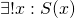is true if there is exactly one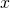in the universe so that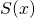is true. We read There is a uniquesuch that."

Notice that mathematically, unique means something rather different from what it means in ordinary English. Mathematically, unique always appears attached to some property. If we said Maxine's a unique person, mathematically we'd mean There is only one person in all the universe, and that one person is Maxine.

Proposition.is equivalent to each of the following:

1.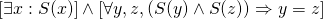2.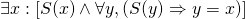Proof.

First we'll show that each of (1) and (2) is enough to guarantee. (1) guarantees that there is at least onewith. If we had two distinct members of the universe, say,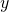and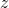, satisfying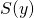and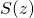, then we'd have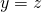, soandwouldn't actually be distinct after all. Thus there is at most onewith.

Now assume (2) is true. We know that there is at least onewith. Suppose we had another, say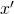. Then applying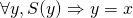with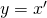, we see that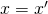. Sowasto begin with. So there is at most onewith.

Now we'll show thatguarantees (1) and (2).

First, examine (1). Since we are proving a statement of the form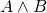, we must establish both conjuncts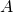and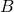. The first conjunct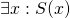is clearly true; there is a uniquewith, therefore there is somewith. Now let's prove the second conjunct,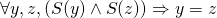. If this were false, we'd have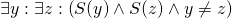. Thus we have two distinct values offor whichis true. But this contradicts.

Now consider (2). We need to find the special; let's use thegiven by. Such anhas. Now givenwith, we see that since there is exactly one valuewith, andand, it must have been that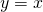.

Thus we've shown thatguarantees (1) and (2) and each of (1) and (2) guarantees. This completes the proof.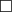We can describe (1) above as saying: there is at least onewith, and there is at most onewith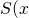).

We can describe (2) as saying: there is a specialwith has both, and the property that wheneveris true,must be the same as.

Here we could say some words about how to prove a statement of the form, but we'll postpone that a bit.

Exercising our powers of what-if-not thinking, let's ask howcould be false. Consider the following statements:

1. There is a unique President of the United States.
2. There is a unique US Senator from Florida.
3. There is a unique US Senator from Washington, DC.

(1) is true. (2) and (3) are false, but false for different reasons. Let's see why:

Exercise. Compute the denial of, using the fact thatcan be expressed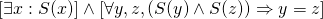Explain the relevance of this to the problem of uniqueness of Senators from Florida and from Washington, DC.